# 长沙综仪生物仪器|器皿清洗机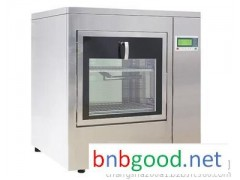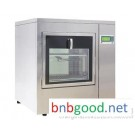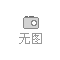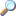点击图片查看原图

 单价： 面议 起订： 1 供货总量： 11 发货期限： 自买家付款之日起 天内发货 所在地： 全球 亚洲 中国 有效期至： 长期有效 最后更新： 2018-02-05 14:45 浏览次数： 996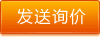===下面的信息是电脑翻译的，请联系发布者
===

r 器皿清洗机概述：
r玻璃器皿清洗机主要用于实验室清洗玻璃器皿、烧杯、试管、载玻片、盖玻片、玻璃吸管、移液管和其他实验室器皿清洗。当被清洗件为精密玻璃器皿、部件或装配件时，玻璃器皿往往成为能满足其特殊技术要求的唯一的清洗方式。r

r

r 产品介绍：
r玻璃器皿清洗机可以对实验室常用实验器皿进行清洗、消毒、干燥全自动处理。主要适用于检验检疫、药厂、化工厂、大学高校、科研所、医院等单位。r

r

r 器皿清洗机性能特点：
r1、整机SUS304不锈钢材质，耐腐蚀、易清洁。
r2、避免实验过程中有害物质对人体的伤害。
r3、实现物品标准化清浩，避免人为清洗不彻底而影响实验效果。
r4、清洗、消毒、干燥一机完成，降低人工成本。
r5、六组固定的清洗程序，预清洗——清洗液清洗——中和清洗——漂洗——热水消毒清洗——干燥
r6、根据器皿不同的污染程度选择不同的清洗程序。r

r

r 可选配件
r1、清洗架手推车，可以与机器完全对接，有效解决人员搬运的难度以及危险；
r2、可选各种不同清洗架（试管、烧杯、培养皿、移液管等4套不同清洗架）；
r3、可配置打印机对清洗过程中的关键信息进行打印、保存；
r4、信息数据输出接口，可对清洗数据进行读取、保存；r

r

r 技术参数
r
1、容积：220L以上；
r2、电源：380V/50Hz；
r3、标配清洗架：2套（由用户自行选择）；
r4、清洗量：根据用户实际配置的清洗架决定。r

rr r r r r r r r r r r r r r r r r r r r r r r r r r r r r r r r r r r r r r r
 r r 型号r r r r 外型尺寸（L*W*H mm）r r r r 有效清洗容积(L)r r r r 电源r r r r 功率（KW）r r r r 最大噪音r r r r 标准清洗r r r r 清洗架r r r r （V）r r r r （dBa）r r r r 时间(min)r r r r r r r r CTLW-120r r r r 1050*740*830r r r r 120r r r r 220V/50Hzr r r r 5r r r r 52r r r r 60r r r r 2套r r r r CTLW-220r r r r 710*680*1750r r r r 220r r r r 220V/50Hzr r r r 6.2r r r r 60r r r r 60r r r r 2套r r
r

r 如果想了解更多关于长沙综仪生物仪器的信息，请点击如下链接：
r器皿清洗机==https://www.zongyi17.com/prodetail37.html
r长沙综仪物仪器清洗灭菌干燥系列==https://www.zongyi17.com/prolist6.html
r长沙综仪生物科技有限公司==https://www.zongyi17.com/r

r

r 长沙综仪生物科技有限公司
r联系电话：0731-81800895/15377480986/15377318545
r业务QQ：154690405
r售后服务：0731-81800895
r公司网址：www.zongyi17.com
r公司地址：湖南长沙开福区万家丽北路一段988号r

rr